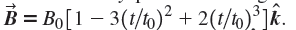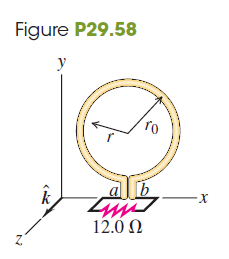Problem

# CALC A circular conducting ring with radius r0 = 0.0420 m lies in the xy-plane in a...

CALC A circular conducting ring with radius r0 = 0.0420 m lies in the xy-plane in a region of uniform magnetic fieldIn this expression, t0 = 0.0100 s and is constant, t is time,is the unit vector in the +z-direction, and B0 = 0.0800 T and is constant. At points a and b (Fig. P29.58) there is a small gap in the ring with wires leading to an external circuit of resistance R = 12.0 Ω. There is no magnetic field at the location of the external circuit. (a) Derive an expression, as a function of time, for the total magnetic flux ΦB through the ring. (b) Determine the emf induced in the ring at time t = 5.00 × 10-3 s. What is the polarity of the emf? (c) Because of the internal resistance of the ring, the current through R at the time given in part (b) is only 3.00 mA. Determine the internal resistance of the ring. (d) Determine the emf in the ring at a time t = 1.21 × 10-2 s. What is the polarity of the emf? (e) Determine the time at which the current through R reverses its direction.#### Step-by-Step Solution

Request Professional Solution

#### Request solution!

We need 10 more requests to produce this problem solution. Share with your friends to get the problem solution faster!

0 /10 have requested this problem solution

Once 10 people have made a request, the problem solutions will be available in 1-2 days.
All students who have requested the problem solution will be notified once they are available.